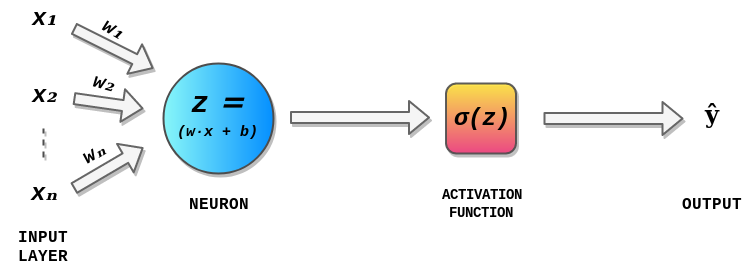# A Gentle Introduction to Math Behind Neural Networks

Source: Deep Learning on Medium# A Gentle Introduction To Math Behind Neural Networks

## Math Concepts Behind Neural Networks From Scratch

Today, with open source machine learning software libraries such as TensorFlow, Keras or PyTorch we can create neural network, even with a high structural complexity, with just a few lines of code. Having said that, the Math behind neural networks is still a mystery to some of us and having the Math knowledge behind neural networks and deep learning can help us understand what’s happening inside a neural network. It is also helpful in architecture selection, fine-tuning of Deep Learning models, hyperparameters tuning and optimization.

# Introduction

I ignored understanding the Math behind neural networks and Deep Learning for a long time as I didn’t have good knowledge of algebra or differential calculus. Few days ago, I decided to to start from scratch and derive the methodology and Math behind neural networks and Deep Learning, to know how and why they work. I also decided to write this article, which would be useful to people like me, who finds it difficult to understand these concepts.

# Perceptrons

Perceptrons — invented by Frank Rosenblatt in 1957, are the simplest neural network that consist of n number of inputs, only one neuron and one output, where n is the number of features of our dataset. The process of passing the data through the neural network is know as forward propagation and the forward propagation carried out in a Perceptron is explained in the following three steps.

Step 1 : For each input, multiply the input value xᵢ with weights wᵢ and sum all the multiplied values. Weights — represent the strength of the connection between neurons and decides how much influence the given input will have on the neuron’s output. If the weight w₁ has higher value than the weight w₂, then the input x₁ will have higher influence on the output than w₂.